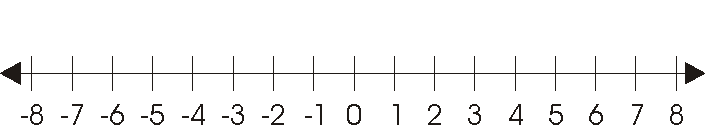Opposite Numbers

Every number except for zero has an opposite. To find the opposite of a number just replace the sign with its opposite. Examples of numbers and their opposites are: 6 and -6,     -32 and 32,     -5.3 and 5.3. (Numbers that are opposites are the same distance from zero, one is on the positive side of zero and the other is on the negative side of zero. They both have the same absolute value, they are just on opposite sides of zero.)